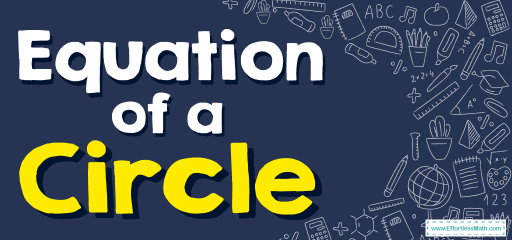# How to Find Equation of a Circle? (+FREE Worksheet!)

The equation of a circle provides an algebraic way to describe a circle and in this article, we teach you how to write two forms of the Equation of a Circle.## Rules for Equation of a Circle

• Equation of circles in standard form: $$(x- h)^2+( y-k)^2= r^2$$
Center: $$h,k$$, Radius: $$r$$
• Equation of circles in general form: $$x^2+y^2+Ax+By+C=0$$

### Equation of a Circle – Example 1:

Write the standard form equation of each circle.
$$x^2+ y^2-4x-6y+9=0$$

Solution:

The standard form of circle equation is: $$(x- h)^2+( y-k)^2= r^2$$
where the radius of the circle is $$r$$, and it’s centered at $$h,k$$.
First, move the loose number to the right side: $$x^2+ y^2-4x-6y=-9$$
Group $$x$$-variables and $$y$$-variables together: $$(x^2-4x)+(y^2-6y)=-9$$
Convert $$x$$ to square form: $$(x^2-4x+4)+y^2-6y=-9+4→(x-2)^2+(y^2-6y)=-9+4$$
Convert $$y$$ to square form: $$(x-2)^2+(y^2-6y+9)=-9+4+9→(x-2)^2+(y-3)^2=4$$
Then:$$(x-2)^2+(y-3)^2=2^2$$

### Equation of a Circle – Example 2:

Write the standard form equation of this circle.
$$x^2+ y^2+6x-10y-2=0$$

Solution:

$$(x- h)^2+( y-k)^2= r^2$$ is the circle equation with a radius $$r$$, centered at $$h,k$$. To find this equation, first, move the loose number to the right side: $$x^2+ y^2+6x-10y=2$$
Group $$x$$-variables and $$y$$-variables together: $$(x^2+6x)+(y^2-10y)=2$$
Convert $$x$$ to square form:
$$(x^2+6x+9)+(y^2-10y)=2+9→(x+3)^2+(y^2-10y)=2+9→$$
Convert $$y$$ to square form:
$$(x+3)^2+(y^2-10y+25)=2+9+25→(x+3)^2+(y-5)^2=36$$
Then: $$(x-(-3))^2+(y-5)^2=6^2$$

### Equation of a Circle – Example 3:

Write the standard form equation of this circle.
$$x^2+ y^2+14x-12y+4=0$$

Solution:

$$(x- h)^2+( y-k)^2= r^2$$ is the circle equation with a radius $$r$$, centered at $$h,k$$. To find this equation, first, move the loose number to the right side: $$x^2+ y^2+14x-12y+4=0$$
Group $$x$$-variables and $$y$$-variables together: $$(x^2+14x)+(y^2-12y)=-4$$
Convert $$x$$ to square form:
$$(x^2+14x+49)+(y^2-12y)=-4+49→(x+7)^2+(y^2-12y)=-4+49→$$
Convert $$y$$ to square form:
$$(x+7)^2+(y^2-12y+36)=-4+49+36→(x+7)^2+(y-6)^2=81$$
Then: $$(x-(-7))^2+(y-6)^2=9^2$$

### Equation of a Circle – Example 4:

Write the standard form equation of this circle.
$$x^2+ y^2+18x+2y-18=0$$

Solution:

$$(x- h)^2+( y-k)^2= r^2$$ is the circle equation with a radius $$r$$, centered at $$h,k$$. To find this equation, first, move the loose number to the right side: $$x^2+ y^2+18x+2y=18$$
Group $$x$$-variables and $$y$$-variables together: $$(x^2+18x)+(y^2+2y)=18$$
Convert $$x$$ to square form:
$$(x^2+18x+81)+(y^2+2y)=18+81→(x+9)^2+(y^2+2y)=18+81→$$
Convert $$y$$ to square form:
$$(x+9)^2+(y^2+2y+1)=18+81+1→(x+9)^2+(y+1)^2=100$$
Then: $$(x-(-9))^2+(y-(-1))^2=10^2$$

## Exercises for Equation of a Circle

### Write the standard form equation of each circle.

1. $$\color{blue}{x^2+y^2-2x+2y=7}$$
2. $$\color{blue}{x^2+y^2+12x+8y=-3}$$
3. $$\color{blue}{x^2+y^2+8x-2y=8}$$
4. $$\color{blue}{x^2+y^2-4x-8y-4=0}$$
5. $$\color{blue}{Center: (2,-4), Radius: 5}$$
6. $$\color{blue}{Center: (-1,-5), Area: 16π}$$
1. $$\color{blue}{(x-1)^2+(y-(-1))^2=3^2}$$
2. $$\color{blue}{(x-(-6))^2+(y-(-4))^2=7^2}$$
3. $$\color{blue}{(x-(-4))^2+(y-1)^2=5^2}$$
4. $$\color{blue}{(x-2)^2+(y-4)^2=(2\sqrt{6})^2}$$
5. $$\color{blue}{(x-2)^2+(y-(-4))^2=5^2}$$
6. $$\color{blue}{(x-(-1))^2+(y-(-5))^2=4^2}$$

### What people say about "How to Find Equation of a Circle? (+FREE Worksheet!) - Effortless Math: We Help Students Learn to LOVE Mathematics"?

No one replied yet.

X
30% OFF

Limited time only!

Save Over 30%

SAVE $5 It was$16.99 now it is \$11.99